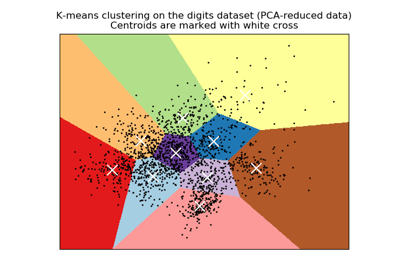/scikit-learn

# sklearn.preprocessing.scale

`sklearn.preprocessing.scale(X, axis=0, with_mean=True, with_std=True, copy=True)` [source]

Standardize a dataset along any axis

Center to the mean and component wise scale to unit variance.

Read more in the User Guide.

Parameters: `X : {array-like, sparse matrix}` The data to center and scale. `axis : int (0 by default)` axis used to compute the means and standard deviations along. If 0, independently standardize each feature, otherwise (if 1) standardize each sample. `with_mean : boolean, True by default` If True, center the data before scaling. `with_std : boolean, True by default` If True, scale the data to unit variance (or equivalently, unit standard deviation). `copy : boolean, optional, default True` set to False to perform inplace row normalization and avoid a copy (if the input is already a numpy array or a scipy.sparse CSC matrix and if axis is 1).

`StandardScaler`
Performs scaling to unit variance using the``Transformer`` API (e.g. as part of a preprocessing `sklearn.pipeline.Pipeline`).

#### Notes

This implementation will refuse to center scipy.sparse matrices since it would make them non-sparse and would potentially crash the program with memory exhaustion problems.

Instead the caller is expected to either set explicitly `with_mean=False` (in that case, only variance scaling will be performed on the features of the CSC matrix) or to call `X.toarray()` if he/she expects the materialized dense array to fit in memory.

To avoid memory copy the caller should pass a CSC matrix.

NaNs are treated as missing values: disregarded to compute the statistics, and maintained during the data transformation.

For a comparison of the different scalers, transformers, and normalizers, see examples/preprocessing/plot_all_scaling.py.

## Examples using `sklearn.preprocessing.scale`A demo of K-Means clustering on the handwritten digits data